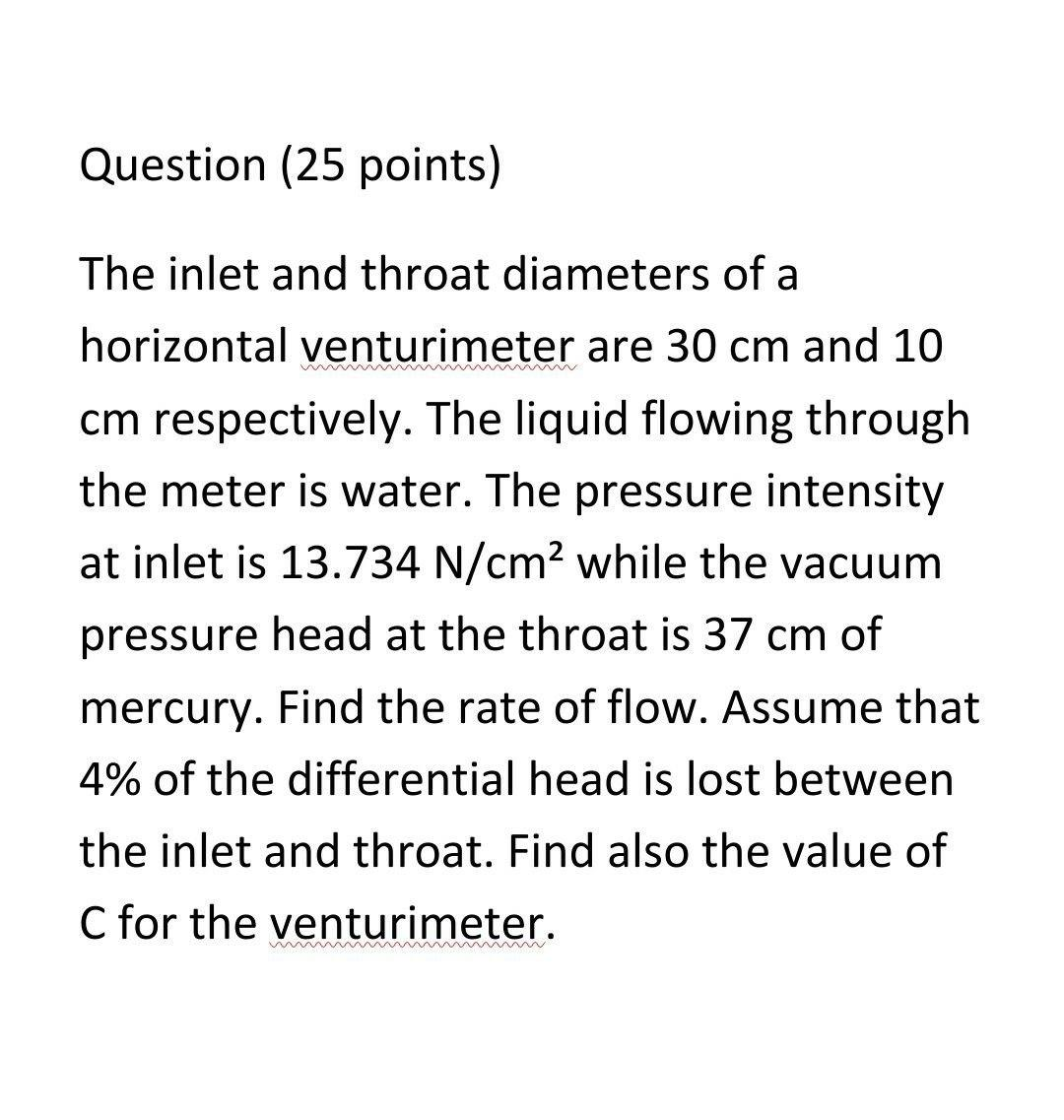# The inlet and throat diameters of a horizontal venturimeter are 30 cm and 10 cm respectively. The liquid flowing through the meter is water. The pressure intensity at inlet is 13.734 N/cm2 while the vacuum pressure head at the throat is 37 cm of mercury. Find the rate of flow. Assume that 4% of the differential head is lost between the inlet and throat. Find also the value of C for the venturimeter

Question-AnswerCategory: Fluid MechanicsThe inlet and throat diameters of a horizontal venturimeter are 30 cm and 10 cm respectively. The liquid flowing through the meter is water. The pressure intensity at inlet is 13.734 N/cm2 while the vacuum pressure head at the throat is 37 cm of mercury. Find the rate of flow. Assume that 4% of the differential head is lost between the inlet and throat. Find also the value of C for the venturimeter

The inlet and throat diameters of a horizontal venturimeter are 30 cm and 10 cm respectively. The liquid flowing through the meter is water. The pressure intensity at inlet is 13.734 N/cm2 while the vacuum pressure head at the throat is 37 cm of mercury. Find the rate of flow. Assume that 4% of the differential head is lost between the inlet and throat. Find also the value of C for the venturimeter